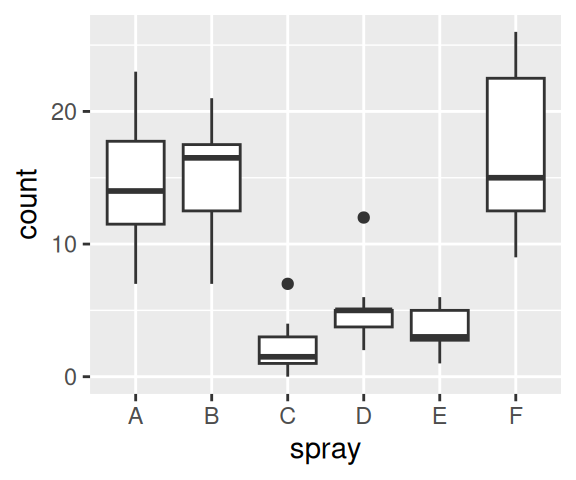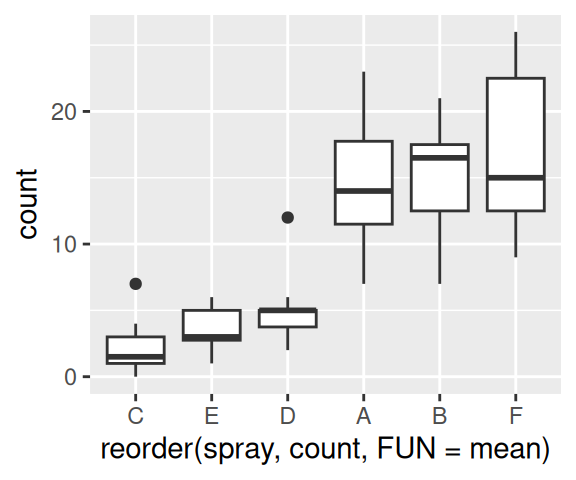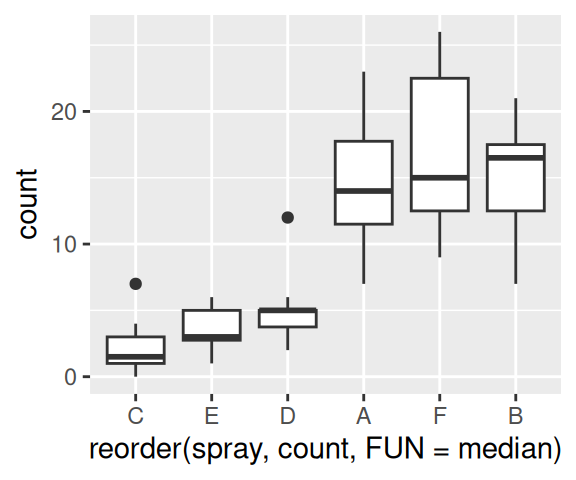## 15.9 Changing the Order of Factor Levels Based on Data Values

### 15.9.1 Problem

You want to change the order of levels in a factor based on values in the data.

### 15.9.2 Solution

Use `reorder()` with the factor that has levels to reorder, the values to base the reordering on, and a function that aggregates the values:

``````# Make a copy of the InsectSprays data set since we're modifying it
iss <- InsectSprays
iss\$spray
#>   A A A A A A A A A A A A B B B B B B B B B B B B C C C C C C C C C C C C
#>  D D D D D D D D D D D D E E E E E E E E E E E E F F F F F F F F F F F F
#> Levels: A B C D E F

iss\$spray <- reorder(iss\$spray, iss\$count, FUN = mean)
iss\$spray
#>   A A A A A A A A A A A A B B B B B B B B B B B B C C C C C C C C C C C C
#>  D D D D D D D D D D D D E E E E E E E E E E E E F F F F F F F F F F F F
#> attr(,"scores")
#>         A         B         C         D         E         F
#> 14.500000 15.333333  2.083333  4.916667  3.500000 16.666667
#> Levels: C E D A B F``````

Notice that the original levels were `ABCDEF`, while the reordered levels are `CEDABF`. What we’ve done is reorder the levels of `spray` based on the mean value of `count` for each level of `spray`.

### 15.9.3 Discussion

The usefulness of `reorder()` might not be obvious from just looking at the raw output. Figure 15.1 shows three plots made with `reorder()`. In these plots, the order in which the items appear is determined by their values.Figure 15.1: Original data (left); Reordered by the mean of each group (middle); Reordered by the median of each group (right)

In the middle plot in Figure 15.1, the boxes are sorted by the mean. The horizontal line that runs across each box represents the median of the data. Notice that these values do not increase strictly from left to right. That’s because with this particular data set, sorting by the mean gives a different order than sorting by the median. To make the median lines increase from left to right, as in the plot on the right in Figure 15.1, we used the `median()` function in `reorder()`.

The tidyverse function for reordering factors is `fct_reorder()`, and it is used the same way as `reorder()`. These do the same thing:

``````reorder(iss\$spray, iss\$count, FUN = mean)
fct_reorder(iss\$spray, iss\$count, .fun = mean)``````